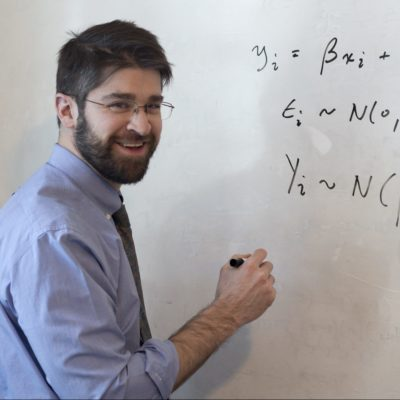# Math Class

The Infinite in Mathematics

Mathematics routinely deals with infinitely large sets of objects.  At first, it may seem rather implausible that we could say much of anything very definite about such fundamentally indefinite collections.  How, for example, can we know something about all integers, when nearly all integers are much larger than we could possibly comprehend?  In this short course, we’ll investigate several methods for exploring the infinite, as well as their fascinating and sometimes counterintuitive results.

1. Introduction, Infinity of Primes and the Sparsity of Primes, Some “Big” Numbers

2. Proofs about the Natural Numbers, Mathematical Induction

3. Fundamental Concepts in the Mathematical Analysis of Infinity

4. The Denumerability of the Rationals

5. The Non-Denumerability of the Reals

## Taught by: